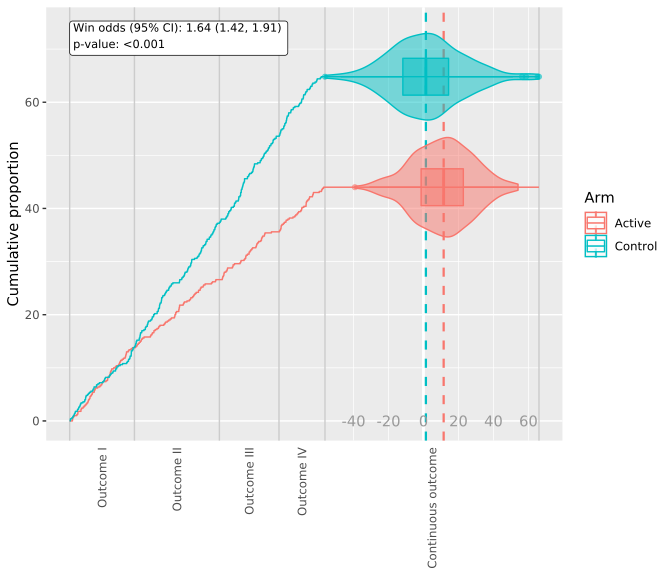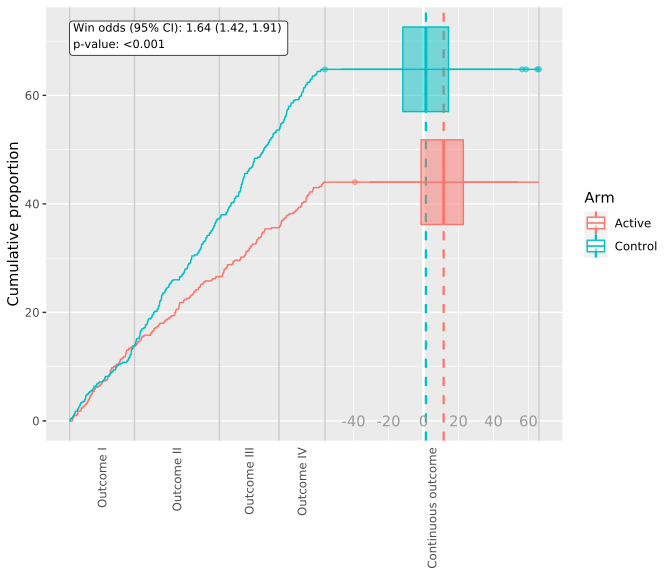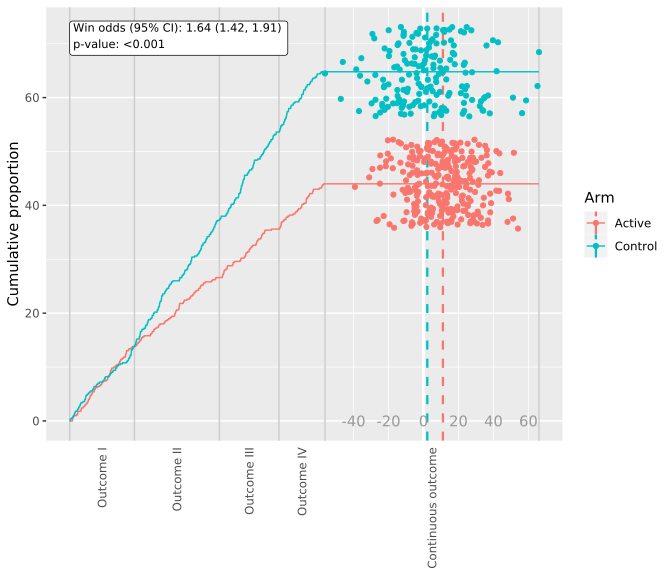# Introduction

Hierarchical composite endpoints (HCE) are complex endpoints combining events of different clinical importance into an ordinal outcome that prioritize the most severe event of a patient. Up to now, one of the difficulties in interpreting HCEs has been the lack of proper tools for visualizing the treatment effect captured by HCE. This package makes it possible to visualize endpoints consisting of the combination of one to several time-to-event (TTE) outcomes (like Death, Hospitalization for heart failure, …) with one continuous outcome (like a symptom score).

The maraca plot captures all important features of how the HCE measures the treatment effect: 1. the percentage of time-to-event outcomes in the overall population, 2. the treatment effect on the combined and individual time-to-event outcomes, and 3. the treatment effect on the continuous component.

# Basic usage

Plotting with maraca requires to have the data in an appropriate format. We provide a few scenarios in the package, named `hce_scenario_a`, `hce_scenario_b`, `hce_scenario_c` and `hce_scenario_d`. These scenarios cover four cases: a) Treatment effect is driven by a combination of TTE outcomes and continuous outcome, b) No treatment effect on neither the TTE outcomes nor on the continuous outcome, c) A large treatment effect on time-to-event outcomes, but no effect on continuous outcome, and finally d) A small treatment effect on time-to-event outcomes, with a larger effect on continuous outcomes, respectively. Other HCE scenarios can easily be simulated with the hce package. The hce package is also used for win odds calculations in the maraca package.

``````library(maraca)

data(hce_scenario_a, package = "maraca")

data <- hce_scenario_a
##   X SUBJID              GROUP GROUPN      AVAL0       AVAL    TRTP
## 1 1      1          Outcome I      0 120.440921   120.4409  Active
## 2 2      2 Continuous outcome  40000   3.345229 40003.3452 Control
## 3 3      3 Continuous outcome  40000  22.802615 40022.8026  Active
## 4 4      4          Outcome I      0 577.311386   577.3114 Control
## 5 5      5         Outcome II  10000 781.758081 10781.7581  Active
## 6 6      6        Outcome III  20000 985.097981 20985.0980 Control``````

The `data` data frame contains information on various columns. These columns may have arbitrary names, so the `maraca` function allows you to specify these names and how they map to the internal nomenclature using the `column_names` parameter. This parameter is a named character vector, mapping the standard names used by maraca with the column names in your dataset.

``````column_names <- c(
outcome = "GROUP",
arm = "TRTP",
value = "AVAL0"
)``````

the `outcome` column must be a character column containing the outcome for each entry.

``````unique(data[["GROUP"]])
##  "Outcome I"          "Continuous outcome" "Outcome II"
##  "Outcome III"        "Outcome IV"``````

The strings associated to each entry are arbitrary, so maraca allows you to specify them in the `tte_outcomes` and `continuous_outcome` parameters. Make sure to specify the TTE outcomes in the correct order, starting from the most severe outcome to the least severe outcome. There can only be one continuous outcome.

``````tte_outcomes <- c(
"Outcome I", "Outcome II", "Outcome III", "Outcome IV"
)
continuous_outcome <- "Continuous outcome"``````

the `arm` column must also be a character column describing to which arm each row belongs.

``````unique(data[["TRTP"]])
##  "Active"  "Control"``````

The strings associated to each entry are arbitrary, so maraca allows you to specify them in the `arm_levels` parameter, as a named vector of character strings. In this example, our file contains “Active” and “Control” as identifiers, as seen in the output above, so we will specify

``arm_levels = c(active = "Active", control = "Control")``

Finally, the `original` column must contain numerical values.

We also need to specify the follow-up time for the time-to event outcomes. Note that there can be no observed events in the data after the follow-up time specified.

All of the above can be aggregated in the call to create a maraca object from the given dataset

``````mar <- maraca(
data, tte_outcomes, continuous_outcome, arm_levels, column_names,
fixed_followup_days = 3*365,
compute_win_odds = TRUE
)``````

The win odds can be calculated and will be annotated in the maraca plot if available. However, it is not calculated by default. Please set the `compute_win_odds = TRUE` to make the calculation. The resulting estimate, 95% confidence levels and p-value will be shown in the maraca object and can also be retrieved with

``````mar\$win_odds
##     estimate        lower        upper      p-value
## 1.643265e+00 1.416117e+00 1.906848e+00 9.354073e-12``````

# Plotting the resulting object

To create a maraca plot, simply pass the maraca object as the first argument to the `plot` function. In addition to the maraca object, the user can optionally supply input parameters for selecting the `continuous_grid_spacing`; transforming the x-axis using “sqrt” in the `trans` argument; selecting different representations of the continuous distribution density in the `density_plot_type`; and setting `vline_type` to “mean” or “median”.

The following types of plots that are available: “default”, “violin”, “box”, “scatter”. The default type is just a nested combination of violin and box plot. Figure 1A-D in reference 1 below, can be recreated through applying the maraca function with subsequent plotting on each of the scenario A-D datasets provided in this package. As an example scenario A is shown in the following plot.

``plot(mar, continuous_grid_spacing_x = 20)````plot(mar, continuous_grid_spacing_x = 20, density_plot_type = "box")````plot(mar, continuous_grid_spacing_x = 20, density_plot_type = "scatter", vline_type = "mean")``The plot_maraca function returns a ggplot2 object. This function will not render the plot immediately so you will have to print() it. Conveniently, you can customize your maraca plot with the comprehensive toolbox of ggplot2. For example:

``````p <- plot_maraca(mar, continuous_grid_spacing_x = 20, density_plot_type = "scatter", vline_type = "mean")
p +
theme_bw() + scale_color_manual(values=c("#999999", "#E69F00")) +
theme(axis.text.x.bottom = element_text(vjust = 0.5, hjust = 0.5))``````